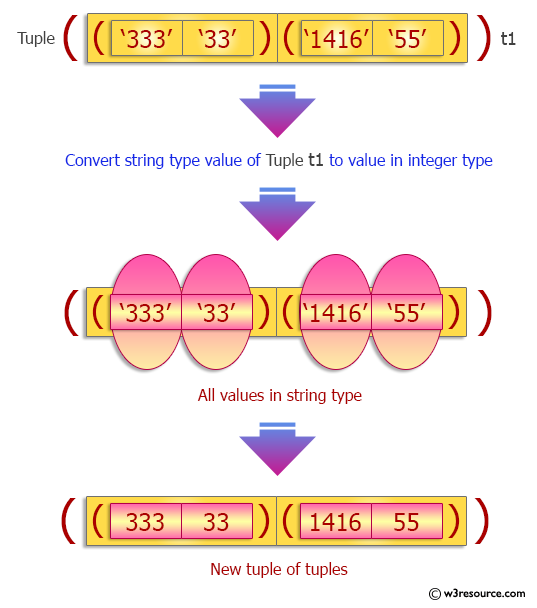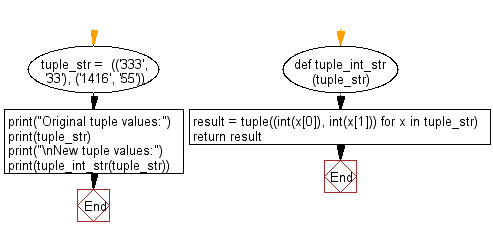﻿ Python: Convert a tuple of string values to a tuple of integer values - w3resource# Python Exercise: Convert a tuple of string values to a tuple of integer values

## Python tuple: Exercise-28 with Solution

Write a Python program to convert a tuple of string values to a tuple of integer values.

Sample Solution:-

Python Code:

``````def tuple_int_str(tuple_str):
result = tuple((int(x), int(x)) for x in tuple_str)
return result

tuple_str =  (('333', '33'), ('1416', '55'))
print("Original tuple values:")
print(tuple_str)
print("\nNew tuple values:")
print(tuple_int_str(tuple_str))
```
```

Sample Output:

```Original tuple values:
(('333', '33'), ('1416', '55'))

New tuple values:
((333, 33), (1416, 55))
```

Pictorial Presentation:Flowchart:## Visualize Python code execution:

The following tool visualize what the computer is doing step-by-step as it executes the said program:

Python Code Editor:

Have another way to solve this solution? Contribute your code (and comments) through Disqus.

What is the difficulty level of this exercise?

Test your Programming skills with w3resource's quiz.

﻿

## Python: Tips of the Day

Decapitalizes the first letter of a string:

Example:

```def tips_decapitalize(s, upper_rest=False):
return s[:1].lower() + (s[1:].upper() if upper_rest else s[1:])
print(tips_decapitalize('PythonTips'))
print(tips_decapitalize('PythonTips', True))
```

Output:

```pythonTips
pYTHONTIPS
```